Updating search results...

# 7 Results

View
Selected filters: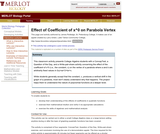Conditional Remix & Share Permitted
CC BY-NC-SA
Rating
0,0 stars

This classroom activity presents College Algebra students with a ConcepTest, a Question of the Day, and a Write-pair-share activity concerning the effect of the coefficient of x^0 (i.e., the constant, c) on the vertex of a parabola where a and b are arbitrarily fixed values in f(x)=ax^2+bx+c.

نوع المادة:
Activity/Lab
التقييم
Provider:
Science Education Resource Center (SERC) at Carleton College
Provider Set:
نقطة البداية (مركز الموارد لتعليم العلوم SERC)
المؤلف:
James J. Rutledge
08/28/2012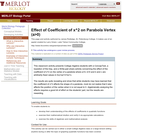Conditional Remix & Share Permitted
CC BY-NC-SA
Rating
0,0 stars

This classroom activity presents College Algebra students with a ConcepTest, a Question of the Day, and a Write-pair-share activity concerning the effect of the coefficient of x^2 on the vertex of a parabola where a>0, b>0 and b and c are fixed values in f(x)=ax^2+bx+c.

نوع المادة:
Activity/Lab
التقييم
Provider:
Science Education Resource Center (SERC) at Carleton College
Provider Set:
نقطة البداية (مركز الموارد لتعليم العلوم SERC)
المؤلف:
James J. Rutledge
08/28/2012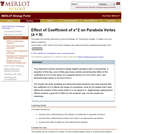Conditional Remix & Share Permitted
CC BY-NC-SA
Rating
0,0 stars

This classroom activity presents College Algebra students with a ConcepTest, a Question of the Day, and a Write-pair-share activity concerning the effect of the coefficient of x^2 on the vertex of a parabola where a<0, b>0 and b and c are fixed values in f(x)=ax^2+bx+c.

نوع المادة:
Activity/Lab
التقييم
Provider:
Science Education Resource Center (SERC) at Carleton College
Provider Set:
نقطة البداية (مركز الموارد لتعليم العلوم SERC)
المؤلف:
James J. Rutledge
08/28/2012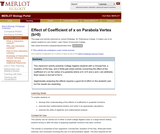Conditional Remix & Share Permitted
CC BY-NC-SA
Rating
0,0 stars

This classroom activity presents College Algebra students with a ConcepTest, a Question of the Day, and a Write-pair-share activity concerning the effect of the coefficient of x on the vertex of a parabola where a>0, b>0 and a and c are fixed values in f(x)=ax^2+bx+c.

نوع المادة:
Activity/Lab
التقييم
Provider:
Science Education Resource Center (SERC) at Carleton College
Provider Set:
نقطة البداية (مركز الموارد لتعليم العلوم SERC)
المؤلف:
James J. Rutledge
08/28/2012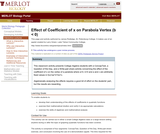Conditional Remix & Share Permitted
CC BY-NC-SA
Rating
0,0 stars

This classroom activity presents College Algebra students with a ConcepTest, a Question of the Day, and a Write-pair-share activity concerning the effect of the coefficient of x on the vertex of a parabola where a>0, b<0 and a and c are fixed values in f(x)=ax^2+bx+c.

نوع المادة:
Activity/Lab
التقييم
Provider:
Science Education Resource Center (SERC) at Carleton College
Provider Set:
نقطة البداية (مركز الموارد لتعليم العلوم SERC)
المؤلف:
James J. Rutledge
08/28/2012Only Sharing Permitted
CC BY-NC-ND
Rating
0,0 stars

This lesson unit is intended to help teachers assess how well students are able to understand what the different algebraic forms of a quadratic function reveal about the properties of its graphical representation. In particular, the lesson will help teachers identify and help students who have the following difficulties: understanding how the factored form of the function can identify a graphŐs roots; understanding how the completed square form of the function can identify a graphŐs maximum or minimum point; and understanding how the standard form of the function can identify a graphŐs intercept.

نوع المادة:
التقييم
Lesson Plan
Provider:
Shell Center for Mathematical Education
Provider Set:
Mathematics Assessment Project (MAP)
04/26/2013قراءة النص المطبوع بخط رفيع
Rating
0,0 stars

This lesson focuses on students making decisions about what tools to apply to solve different problems related to quadratic expressions and equations. It is also intended to build awareness of the form an answer will take in order to help students make sense of the kind of problem they are solving. (9th/10th/11th Grade Math)

نوع المادة:
Activity/Lab
Lecture
Lesson Plan
Teaching/Learning Strategy
Provider:
Noyce Foundation
Provider Set:
في قلب الرياضيات
المؤلف:
Shreve, Barbara